THE PYTHAGOREAN THEOREMLearning Objectives - This is what you must know after studying the lecture and doing the practice problems!

1.   Memorize and apply the Square Root Property
2.   Memorize and apply the Pythagorean TheoremThe Square Root Property

The Square Root Property is most often used when solving certain quadratic equations. Specifically, those of the form ax2 = c where a cannot be equal to 0, but c can be equal to 0.

The Square Root Property states the following:

If u is an algebraic expression containing a variable and d is a constant, thenhas exactly two solutions, namelyandor simply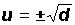.

Example 1:

Solve the quadratic equation.

First, we isolate the squared term to get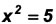Now, we use the Square Root Property. In this case, the x in the equation is the u in the formulaand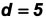.

That is,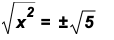then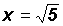and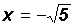orNote that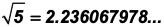when evaluated with a calculator. There are infinitely many decimal places which means that we are dealing with an irrational number.

Example 2:

Solve the quadratic equation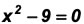.

First, we isolate the squared term to get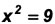.

Then we use the Square Root Property.

That is,then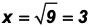and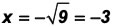or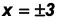Please note that we have an integer solution.

The Pythagorean Theorem

The right triangle is associated with one of the most famous and useful theorems (assertions that can be proved true using the rules of logic) in mathematics. It is called the Pythagorean Theorem named for the Greek mathematician Pythagoras.

The Pythagorean Theorem is probably one of the most important mathematical equation used in the building trade. Due to is, drawings can be enlarged, foundations can be build, and square angles can be calculated. It can be used by everyone from surveyors who want to find out how tall a mountain is to astronomers who want to calculate the distance to the sun or the circumferences of the moon, to weathermen who try to calculate the height of clouds. Carpenters will use it to keep their work square and draftsmen use it to ensure the accuracy of their architectural drawings.

The Pythagorean Theorem states that the square of the hypotenuse of a right triangle is equal to the sum of the squares of the two legs of the triangle.The Pythagorean Theorem is usually presented as follows:, where aand b are the lengths of the legs of a right triangle and c is the length of its hypotenuse.

Example 3:

Given a right triangle, assume that the lengths of the legs are a = 6 and b = 7.5.  Find the length of the hypotenuse c.  Round the answer to one decimal place.

Using the Pythagorean Theorem, we get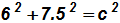We are now dealing with a quadratic equation where c is the variable. We use the Square Root Property to getNOTE that we are not using the negative solution as shown in Examples 1 and 2 since the sides of triangles are never negative.

Using the calculator, we findThere are infinitely many decimal places which means that we are dealing with an irrational number.

Example 4:

Given a right triangle, assume that the length of the leg b is 4 and the length of the hypotenuse c is 5. Find the length of leg a.

Using the Pythagorean Theorem, we get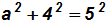We are now dealing with a quadratic equation where a is the variable. We isolated the variable to get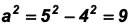Finally, using the Square Root Property we get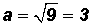NOTE that we are not using the negative solution as shown in Example 1 and 2 since the sides of triangles are never negative.